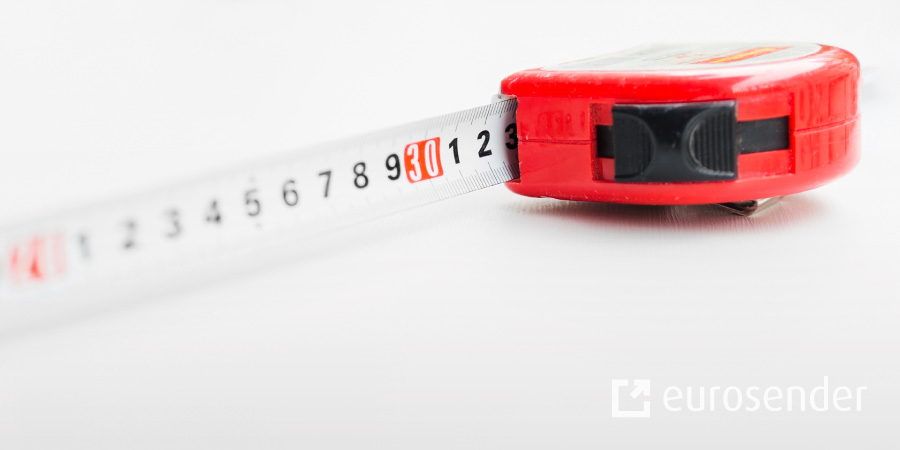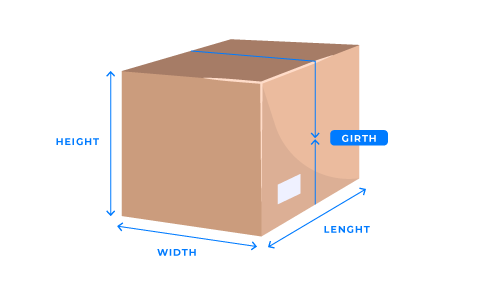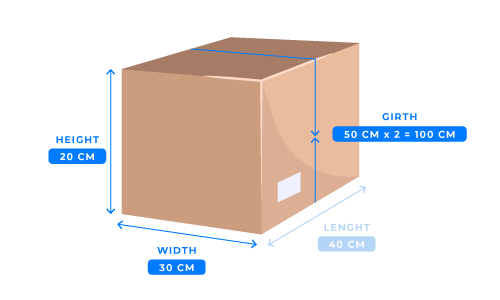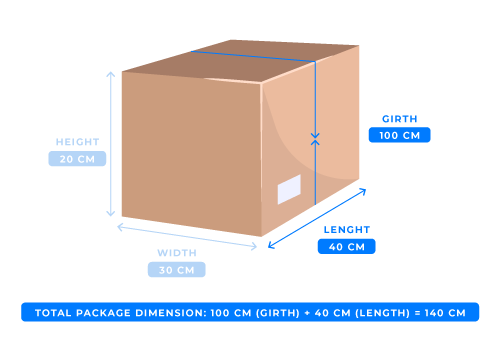How to Calculate the Girth and Length of a Package?4 min readMany logistics companies have specific requirements when it comes to the weight, length or girth of a package, and when shipping, they ask customers to be as precise as possible. But how can you measure the girth of a package? Continue reading and find out!

What is the girth of a box?

How to measure the girth of a box?

How to measure the combined length and girth of a box?

Calculating girth of a package – Importance

# What is the girth of a box?

Several factors determine the shipping price, such as the shipping countries, the weight, the length of the parcel, and many more. The girth of a package will be another factor in determining the shipping price. In simplest words, the girth is measuring the distance around the package.

You may also be interested in:

It is important to remember that the length of the parcel is always the longest side of the package! So, the girth of the package will be calculated from the width and height of the widest parcel points.Some calculators automatically determine the price after you insert all the measurements, and you can avoid measuring the package girth or length on your own. And at Eurosender, we have simplified the process for you! With our shipping dimensions tool, you will be able to calculate the shipping price immediately, just after you have inserted the dimensions of your package! Are you curious about how that works? Check the link below!

## How to measure the girth of a box?

Every courier company has different requirements for the girth of a parcel. Measure the girth of a package correctly to avoid unwanted scenarios such as getting a surcharge, returned parcel or even a failed collection or delivery. Here is the formula on how to calculate the girth of a package:

Step 1: Measure the three dimensions of the box in centimetres. For example:

40 cm (length) x 30 cm (width) x 20 cm (height)

Step 2: To get the girth of the package, add the width and height together and multiply by two. For example:

30 cm (width) + 20 cm (height) = 50 cm.

The girth is: 50 cm x 2 = 100 cm.### How to measure the combined length and girth of a box?

Now that we know how to measure the girth of the box, we can calculate the total package size by, combining the length and girth of the box together.

Step 3: Now, it is time to add the girth and the longest dimension of the parcel together. Here is the formula on how to calculate the length and girth of a box – combined by using the same example from earlier:

From previous steps – The girth was 100 cm. The length was 40 cm.

Total package dimension: 100 cm (girth) + 40 cm (length) = 140 cm (combined length and girth)